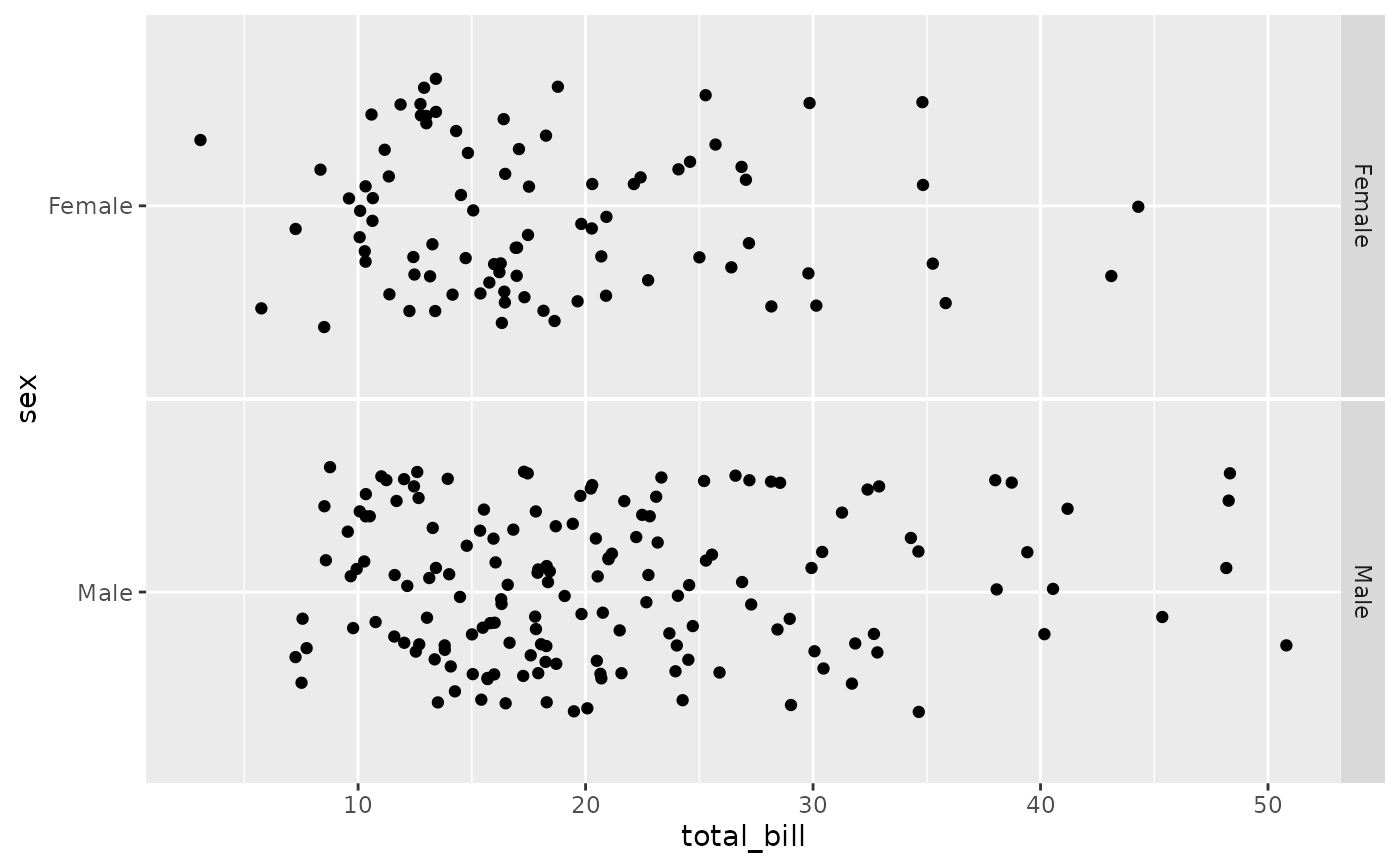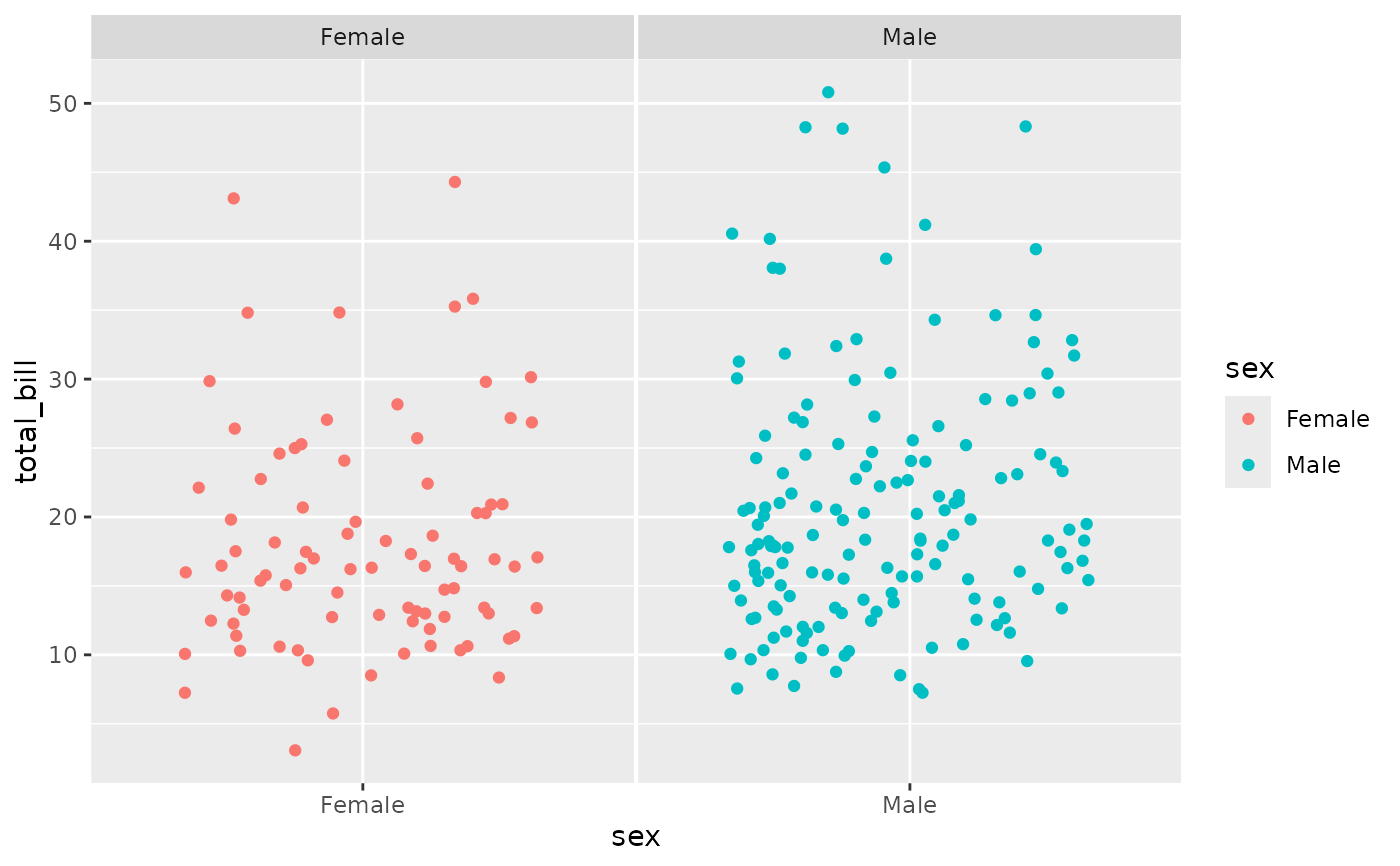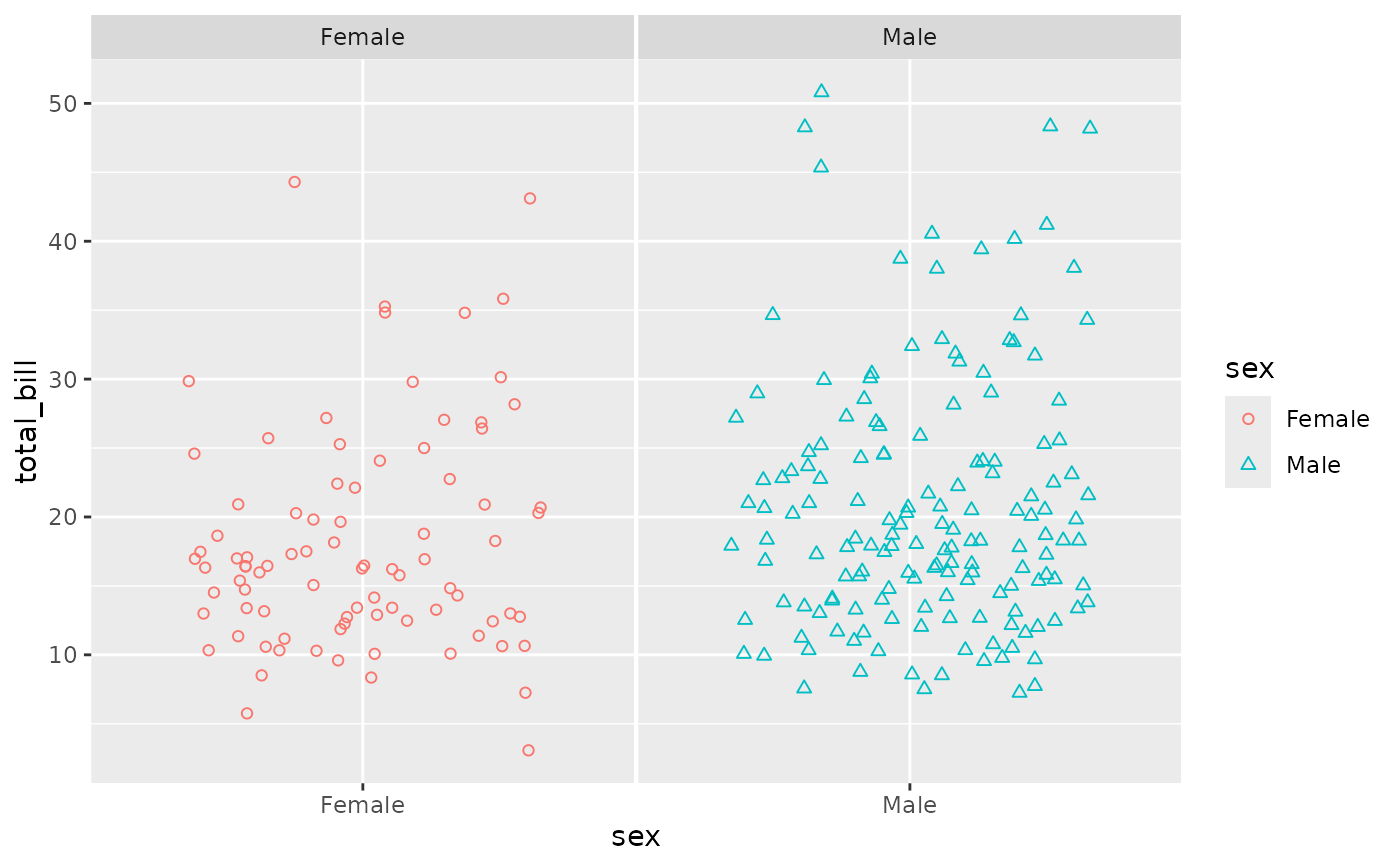Add jittering with the box plot. ggally_dot_no_facet will be a single panel plot, while ggally_dot will be a faceted plot

ggally_dot(data, mapping, ...)

ggally_dot_no_facet(data, mapping, ...)

## Arguments

data data set using aesthetics being used other arguments being supplied to geom_jitter

Barret Schloerke

## Examples

# Small function to display plots only if it's interactive
p_ <- GGally::print_if_interactive

data(tips, package = "reshape")
p_(ggally_dot(tips, mapping = ggplot2::aes(x = total_bill, y = sex)))p_(ggally_dot(tips, mapping = ggplot2::aes_string(x = "total_bill", y = "sex")))p_(ggally_dot(
tips,
mapping = ggplot2::aes_string(y = "total_bill", x = "sex", color = "sex")
))p_(ggally_dot(
tips,
mapping = ggplot2::aes_string(y = "total_bill", x = "sex", color = "sex", shape = "sex")
) + ggplot2::scale_shape(solid=FALSE))